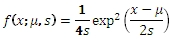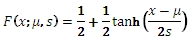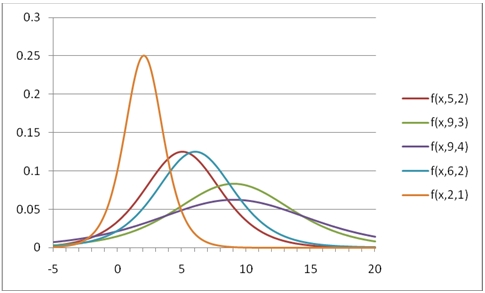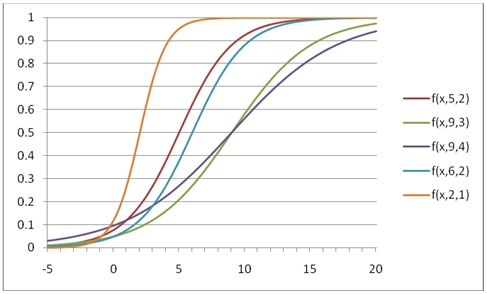# SQL Server Logistic distribution function

LOGISTIC

Updated: 31 July 2010

Use LOGISTIC to calculate the probability density function or the lower cumulative distribution function of the logistic distribution.

The formula for the probability density function is:The lower cumulative distribution function is:Syntax
SELECT [wctStatistics].[wct].[LOGISTIC] (
<@X, float,>
,<@A, float,>
,<@B, float,>
,<@Cumulative, bit,>)
Arguments
@X
is the value to be evaluated. @X is an expression of type float or of a type that implicitly converts to float
@A
is the location parameter. @A is an expression of type float or of a type that implicitly converts to float
@B
is the scale parameter. @B is an expression of type float or of a type that implicitly converts to float
@Cumulative
is a logical value that determines if the probability density function ('False', 0) or the cumulative distribution function ('True', 1) is being calculated. @Cumulative is an expression of type bit or of a type that implicitly converts to bit

Return Types
float
Remarks
·         @B must be greater than zero (@B > 0).
·         The upper cumulative distribution function is equal to 1 –LOGISTIC(@X,@A,@B,'True').
Examples
Calculate the probability density function:
SELECT wct.LOGISTIC(1,0,1,'False')
This produces the following result
----------------------
0.196611933241482

(1 row(s) affected)

You can use the SeriesFloat function from the XLeratorDB/math library to generate a dataset which can be pasted into EXCEL to generate a graph of the probability density function.
SELECT SeriesValue
,wct.LOGISTIC(SeriesValue, 5, 2, 'False') as [f(x,5,2)]
,wct.LOGISTIC(SeriesValue, 9, 3, 'False') as [f(x,9,3)]
,wct.LOGISTIC(SeriesValue, 9, 4, 'False') as [f(x,9,4)]
,wct.LOGISTIC(SeriesValue, 6, 2, 'False') as [f(x,6,2)]
,wct.LOGISTIC(SeriesValue, 2, 1, 'False') as [f(x,2,1)]
FROM wct.SeriesFloat(-5, 20, .1,NULL,NULL)
This is an EXCEL-generated graph of the resultsCalculate the lower cumulative distribution function:
SELECT wct.LOGISTIC(1,0,1,'True')
This produces the following result
----------------------
0.731058578630005

(1 row(s) affected)

You can use the SeriesFloat function from the XLeratorDB/math library to generate a dataset which can be pasted into EXCEL to generate a graph of the cumulative distribution function.
SELECT SeriesValue
,wct.LOGISTIC(SeriesValue, 5, 2, 'True') as [f(x,5,2)]
,wct.LOGISTIC(SeriesValue, 9, 3, 'True') as [f(x,9,3)]
,wct.LOGISTIC(SeriesValue, 9, 4, 'True') as [f(x,9,4)]
,wct.LOGISTIC(SeriesValue, 6, 2, 'True') as [f(x,6,2)]
,wct.LOGISTIC(SeriesValue, 2, 1, 'True') as [f(x,2,1)]
FROM wctMath.wct.SeriesFloat(-5, 20, .1,NULL,NULL)
This is an EXCEL-generated graph of the resultsCalculate the upper cumulative distribution function:
SELECT 1 - wct.LOGISTIC(1,0,1,'True')
This produces the following result
----------------------
0.268941421369995

(1 row(s) affected)

### SupportCopyright 2008-2021 Westclintech LLC         Privacy Policy        Terms of Service